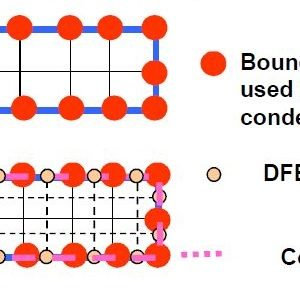﻿ Matrix | Aerospace Engineering

## Static Condensation – FEM AnalysisBackground Theory With “condensation” is indicated a procedure adopted for the structural analysis to carry out a matrix of stiffness and load boundary conditions of a detailed finite model (DFEM), starting from a raw global finite model (GFEM). The DFEM … Continue reading

## 2.3. Laminate Stiffener Matrix

1 . Analysis of the Orthotropic Lamina 1.1. Introduction 1.2. Hooke’s Law 1.3. Relationships between elastic constants and Matrix of Elasticity 1.4. Matrix of elasticity   2. Classical theory of Laminates 2.1. Introduction 2.2. Basic Formulas 2.3. Laminate stiffener matrix 2.4. … Continue reading

## 1.3. Relationships between elastic constants

1 . Analysis of the Orthotropic Lamina 1.1. Introduction 1.2. Hooke’s Law 1.3. Relationships between elastic constants and Matrix of Elasticity 1.4. Matrix of Elasticity   2. Classical theory of Laminates 2.1. Introduction 2.2. Basic Formulas 2.3. Laminate stiffener matrix 2.4. … Continue reading

[kofi]